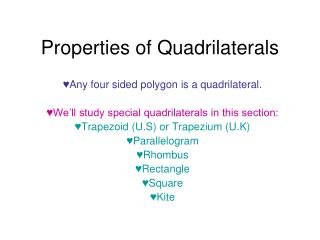DownloadDownload PresentationDownload Presentation- - - - - - - - - - - - - - - - - - - - - - - - - - - E N D - - - - - - - - - - - - - - - - - - - - - - - - - - -
##### Presentation Transcript

1. Properties of Quadrilaterals Any four sided polygon is a quadrilateral. We’ll study special quadrilaterals in this section: Trapezoid (U.S) or Trapezium (U.K) Parallelogram Rhombus Rectangle Square Kite

2. A KITE • 2 pair of consecutive congruent sides • Opposite sides are NOT congruent • Diagonals are perpendicular • Notice only ONE diagonal is bisected

3. Properties of a trapezoid • A trapezoid has one and only one pair of parallel sides.

4. supplementary Properties of parallelograms • Opposite sides of a parallelogram are parallel • Opposite sides are congruent • Opposite angles of a parallelograms are congruent. • Diagonals of a parallelogram bisect each other • Consecutive angles of a parallelogram are supplementary

5. Properties of a Rhombus (Rhombi) • A rhombus is a parallelogram (this means it has ALL of the characteristics of a parallelogram) In addition: • A rhombus has four congruent sides • The diagonals of a rhombus are perpendicular • The diagonals bisect opposite angles

6. Properties of Rectangles • A rectangle is a parallelogram (this means it has ALL the characteristics of a parallelogram) IN ADDITION: • Four right angles • The diagonals of a rectangle are congruent and they bisect each other

7. Properties of Squares • A square is a parallelogram, a rectangle, and a rhombus (It has ALL those characteristics!!!) • Has four congruent sides • Has four right angles • The diagonals of a square: • bisect each other • are congruent • are perpendicular. • Bisect opposite angles

8. Practice Problems. Study Carefully and then take your test. • Is this statement true or false. Explain your answer. • All rhombuses are squares • A rhombus has four congruent sides, but some may or may not have right angles. The statement is false.

9. Is this statement true or false. Explain your answer. • Some trapezoids are squares • A square has two sets of parallel sides, but a trapezoid has only one set of parallel sides. The statement is false.

10. Is this statement true or false. Explain your answer. • Some parallelograms are squares. • A square has all the properties of a parallelogram, so some parallelograms do have equal sides and right angles; however, some do not. The statement is true.

11. Is this statement true or false. Explain your answer • All squares are rectangles • A square has four right angles. A rectangle also has four right angles. So a square is a rectangle. The statement is true.

12. Is this statement true or false. Explain your answer • All rectangles are squares • A square has four right angles and four (2 pairs of) congruent sides. A rectangle has four right angles and 2 (a pair of) congruent sides. The statement is false.

13. Is this statement true or false. Explain your answer • Some rectangles are squares. • If the rectangle has four congruent sides, then it is a square. The statement is true.

14. Classwork • Answer these questions. Give your reasons. • (1.) A quadrilateral with four right angles and four congruent sides is called……………………. • (2.) A quadrilateral with two four right angles and two congruent sides is called……………………….. • (3.) A quadrilateral with exactly one pair of parallel sides is called……………….. • (4.) Name any three quadrilaterals with exactly two pairs of parallel sides • (5.) True or False: All quadrilaterals are parallelograms. • (6.) True or False: Every parallelogram is a rectangle.